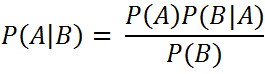Advanced Database Management System - Tutorials and Notes: Simple introduction to Naive Bayes classifier

## Simple introduction to Naive Bayes classifier

### What is Naive Bayes Classifier?

A Naive Bayes classifier is a probabilistic classifier that uses Bayes' theorem with an independence assumption between the features that are used for classification.

### What does Naive Bayes Classifier use?

It uses Bayes' theorem.Here, A is the target class, B is the predictor attribute. P(A|B) is the probability of A given B, i.e. probability of a class given the predictor attribute. Writing this way is called as conditional probability.

What is it used for?

It is used for classification task in machine learning.

### What is independence in Naive Bayes classifier?

A Naive Bayes classifier assumes that the presence of a particular feature in a class is unrelated to the presence of any other feature. For example, to decide playing a game of cricket, the temperature, humidity and wind may be the features. And, temperature may be Hot or Cold, humidity may be High or Low and it may be windy or not. But, these features are not dependent on each other. that means, the Hot temperature is nothing to do with the Low humidity. in simpler terms, each feature is independent and each feature is given equal weight in classification task.

Here is a video lecture from Louis Serrano on Naive Bayes Classifier;